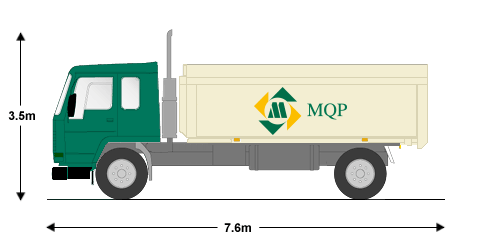# I'm getting 10-15 tonnes of topsoil delivered, what size tarpaulin do I need?

I want to order 10-15 tonnes of top soil.

It'll be delivered by lorry and "tipped" onto the ground. This will make roughly pyramid shape, or perhaps a truncated square pyramid. a cone shape, or a flat topped cone (conical frustrum).Soil repose values: https://structx.com/Soil_Properties_005.html

Topsoil = 35 to 45 repose depending on wetness. (It's not going to be "dry" so 40 feels a good average.)

Standard density for topsoil is around 100 lb/ft3 (1600 kg/m3). ref This other site gives a value of 1250 kg/m3 ref2 but perhaps thats because its "loose" and not defined as 'topsoil'.

The soil should not go straight on the ground, it needs to go on a plastic sheet to cover the ground.

Given this, is it possible the estimate the meters squared area of the base of the shape; to work out how much tarpaulin / plastic sheet is needed?

I don't mind a margin of error up to 1 meter in either direction, if that helps you estimate. :-)

• To answer this accurately you need to estimate the angle of repose for the soil. Soil with a lower angle of repose will require a larger tarpaulin. If this is an actual practical problem you also need to know how accurate the lorry operator is! (If this is the case, have you tried asking the delivery firm how big a tarpaulin they recommend?)
– MJD
Jan 27 at 13:11
• Thank you! I'll go find out what angle the trucks usually tip at. And thanks yeah I did ask the delivery firm first, they just rolled their eyes and said "cover as much of your drive as you need protecting".. not very useful Jan 27 at 13:24
• From my experience, It usually looks like a cone, not a pyramid Jan 27 at 13:27
• @MJD could not find the angle of tip, only the height! (added to the question) Jan 27 at 13:34
• @Vasya ah yes you are right a cone not a pyramid! Jan 27 at 13:35

As mentioned in the comments, the pile will have a cone shape. This is of course assuming that the unloading is done appropriately. The volume of this cone is given by

$$V=\frac13\pi r^2h,$$

where $$h$$ is the height of the cone and $$r$$ is the base radius. With the angle of repose $$\theta_{\mathrm{soil}}$$ we can relate $$r$$ and $$h$$ by

$$h = r \tan(\theta_{\mathrm{soil}}).$$

Using further that the volume $$V$$ taken by a mass $$M$$ of soil is

$$V = \frac{M}{\rho_{\mathrm{soil}}},$$

where $$\rho_{\mathrm{soil}}$$ is the bulk density of soil. So, putting everything together, we arrive at

$$r = \sqrt{ \frac{3M}{\pi\rho_{\mathrm{soil}}\tan(\theta_{\mathrm{soil}})} }.$$

Using the values in your case:

Plugging in that $$M$$ is between $$10000 \mathrm{kg}$$ and $$15000 \mathrm{kg}$$ and common values for the bulk density and angle of response are $$\rho_{\mathrm{soil}}$$ between $$1250\mathrm{kg}/m^3$$ and $$1600 \mathrm{kg}/m^3$$ and $$\theta_{\mathrm{soil}}$$ between $$35°$$ and $$45°$$ (see comments; assuming smaller angles and densities is more conservative), we arrive that the cone will have a base circle radius in the range of

$$r \approx 1.81\mathrm{m}~...~2.54 \mathrm{m}.$$

This range is obtained by using the extremal values of the mentioned ranges.

So, if you want to be on the (very) safe side, you could use a square-shape tarp that has a side length of $$5\mathrm{m}$$ for the pile plus $$1\mathrm{m}$$ margin ($$0.5\mathrm{m}$$ on each side), i.e., a total side length of $$6\mathrm{m}$$ and an area of $$6\mathrm{m}\cdot6\mathrm{m}=36\mathrm{m}^2$$, as displayed in the sketch below.• probably would be good for OP to see largest possible / smallest given the parameter ranges Jan 27 at 14:25
• Again, from my personal experience, go with the highest estimate. I needed 2m by 2m square tarp for 3 cubic yards (very close to cubic meters). Jan 27 at 15:11
• Thanks for this, so I should get a tarp between 1.8m2 to 2.6m2 (and so if I got a 3m2 square one that'll probably cover all eventualities)? Jan 27 at 15:44
• @Blundell Nono. The radius of the base circle is between $1.8$ and $2.5$ meters. So, if you want to use a rectangular (or squared) tarp, it should have side length $2*2.5m=5m$ plus a margin, so maybe $6m$. This gives you a total area of $6m*6m=36m^2$. For a sketch, see here. Note that you might want to adapt margins to your liking! In my post I was computing the radius and area of the pile only! Jan 27 at 16:10
• ah thank you very much! (I was mixing up Radius and Diameter - its been so long 😅) perfect thats extra clear now thanks! Jan 27 at 16:39